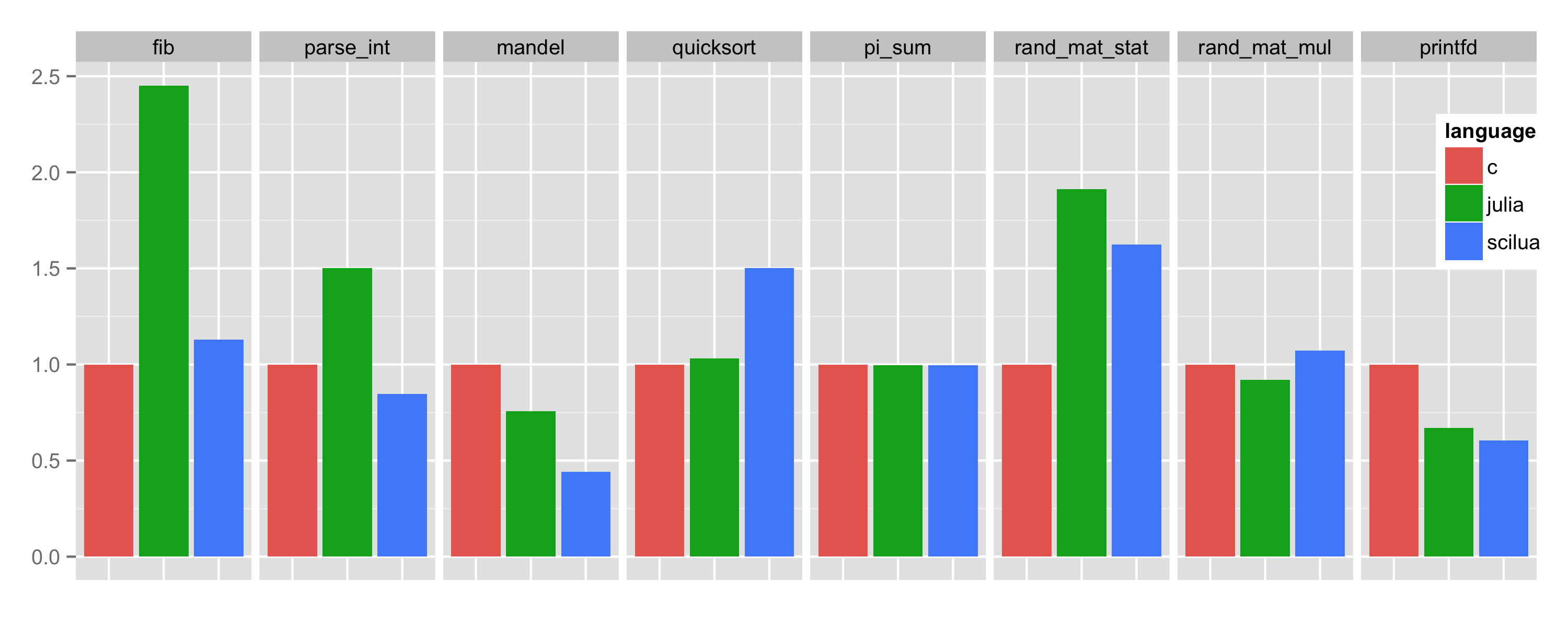scilua.org Go back Open original

# Scientific Computing with LuaJIT

A complete framework for numerical computing based on LuaJIT which combines the ease of use of scripting languages (MATLAB, R, ...) with the high performance of compiled languages (C/C++, Fortran, ...).

Lua is a dynamic language characterized by a small number of simple but powerful concepts that allow to easily implement complex algorithms. Lua is fast to learn thanks to its high-level nature, to the concise syntax and to the precise documentation. Lua is a proven robust language that offers both object-oriented and functional programming features.

LuaJIT is an extremely optimized Just In Time compiler for Lua. Start-up and warm-up times are negligible, compile times are in the microsecond to millisecond range and the typical performance of compiled LuaJIT programs is close to the one of C/C++. LuaJIT includes the FFI library which allows to call C function directly.

## PerformanceThe figure above compares the running time required (lower is better) to run the Julia benchmark suite. Timings are relative to the C implementation.

The details: Linux x64 / gcc 5.2.1 / Julia 0.4.1 / SciLua 1.0 latest, implementation: perf-v1.2.sci.lua.

## Install

The easiest way to install SciLua is via the ULua distribution.

Having installed (i.e. unzipped) ULua, execute:

``````
ulua/bin/upkg add sci # Install the sci package (SciLua).
ulua/bin/upkg add sci-lang # Install the sci-lang package (SciLua syntax extensions).
``````

This is the suggested way as all dependencies are handled by the package manager and SciLua can be kept up to date using `upkg update`.

Alternatively, it is possible to install SciLua manually. Download and unzip sci~latest.zip and sci-lang~latest.zip into folders named respectively `sci` and `sci-lang` and make sure that such modules can be found by LuaJIT (check `package.path` documentation). Notice that SciLua requires xsys and OpenBLAS to be installed as well.

## Sci Library

The `sci` package contains a general purpose scientific computing library composed of the following modules:

Each sub-module has to be loaded separately (`require 'sci'` is not allowed):

``````
-- No global key is set:
local alg = require "sci.alg" -- Load sci.alg module.
local dist = require "sci.dist" -- Load sci.dist module.
``````

## Sci Syntax Extensions

Based on the LuaJIT Language Toolkit, the `sci-lang` package contains the `scilua` executable which extends the syntax of LuaJIT for easier linear algebra operations:

• algebra expressions constructed via empty bracket `[]` indexing
• element-wise operations via plain Lua operators `+-*/^%`
• matrix multiplication via `**`
• matrix exponentiation via `^^`
• transposition via ```
• efficient implementation minimizes required allocations and loops
• support for assignments

For example, the `rand_mat_stat` benchmark shown above is implemented as:

``````
local function randmatstat(t) local n = 5 local v, w = alg.vec(t), alg.vec(t) for i=1,t do local a, b, c, d = randn(n, n), randn(n, n), randn(n, n), randn(n, n) local P = alg.join(a..b..c..d) local Q = alg.join(a..b, c..d) v[i] = alg.trace((P[]`**P[])^^4) -- Matrix transpose, product and power. w[i] = alg.trace((Q[]`**Q[])^^4) -- Matrix transpose, product and power. end return sqrt(stat.var(v))/stat.mean(v), sqrt(stat.var(w))/stat.mean(w)
end
``````